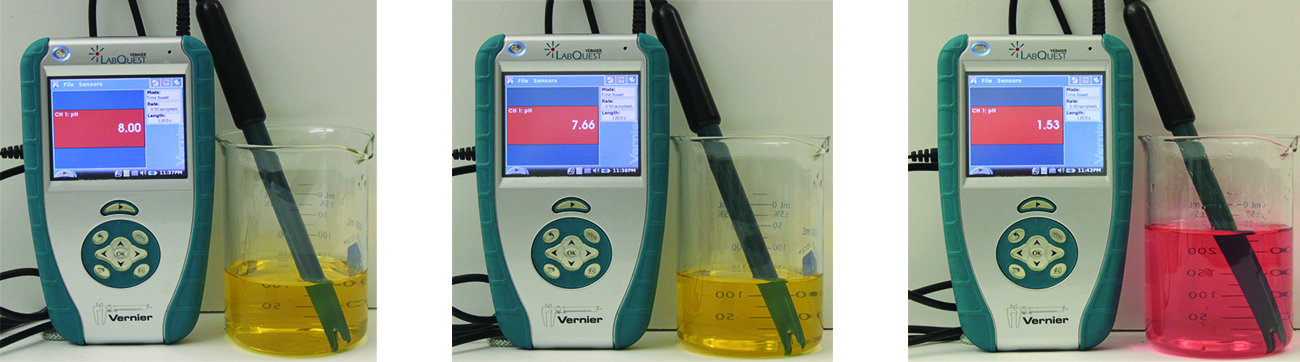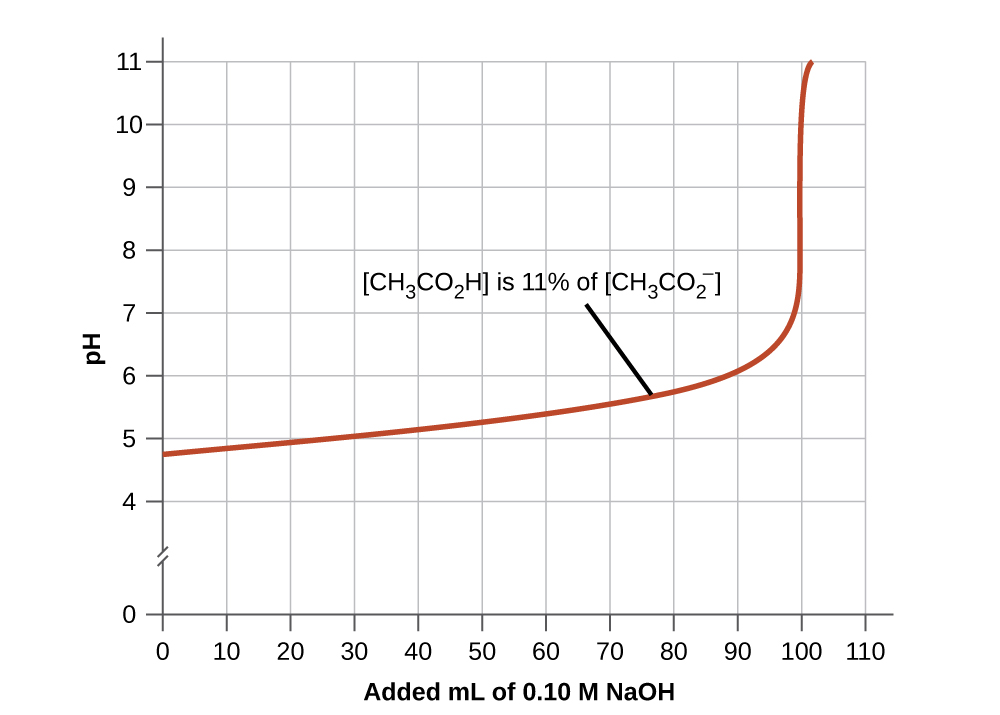14.6 Buffers  (Page 3/9)

 Page 3 / 9
$\left(1.0\phantom{\rule{0.2em}{0ex}}×\phantom{\rule{0.2em}{0ex}}{10}^{-4}\right)\phantom{\rule{0.2em}{0ex}}-\phantom{\rule{0.2em}{0ex}}\left(1.8\phantom{\rule{0.2em}{0ex}}×\phantom{\rule{0.2em}{0ex}}{10}^{-6}\right)\phantom{\rule{0.2em}{0ex}}=9.8\phantom{\rule{0.2em}{0ex}}×\phantom{\rule{0.2em}{0ex}}{10}^{-5}\phantom{\rule{0.2em}{0ex}}M$

The concentration of NaOH is:

$\frac{9.8\phantom{\rule{0.2em}{0ex}}×\phantom{\rule{0.2em}{0ex}}{10}^{-5}\phantom{\rule{0.2em}{0ex}}M\phantom{\rule{0.2em}{0ex}}\text{NaOH}}{0.101\phantom{\rule{0.2em}{0ex}}\text{L}}\phantom{\rule{0.2em}{0ex}}=9.7\phantom{\rule{0.2em}{0ex}}×\phantom{\rule{0.2em}{0ex}}{10}^{-4}M$

The pOH of this solution is:

$\text{pOH}=\text{−log}\left[{\text{OH}}^{\text{−}}\right]\phantom{\rule{0.2em}{0ex}}=\text{−log}\left(9.7\phantom{\rule{0.2em}{0ex}}×\phantom{\rule{0.2em}{0ex}}{10}^{-4}\right)\phantom{\rule{0.2em}{0ex}}=3.01$

The pH is:

$\text{pH}=14.00\phantom{\rule{0.2em}{0ex}}-\phantom{\rule{0.2em}{0ex}}\text{pOH}=10.99$

The pH changes from 4.74 to 10.99 in this unbuffered solution. This compares to the change of 4.74 to 4.75 that occurred when the same amount of NaOH was added to the buffered solution described in part (b).

Show that adding 1.0 mL of 0.10 M HCl changes the pH of 100 mL of a 1.8 $×$ 10 −5 M HCl solution from 4.74 to 3.00.

Initial pH of 1.8 $×$ 10 −5 M HCl; pH = −log[H 3 O + ] = −log[1.8 $×$ 10 −5 ] = 4.74
Moles of H 3 O + in 100 mL 1.8 $×$ 10 −5 M HCl; 1.8 $×$ 10 −5 moles/L $×$ 0.100 L = 1.8 $×$ 10 −6
Moles of H 3 O + added by addition of 1.0 mL of 0.10 M HCl: 0.10 moles/L $×$ 0.0010 L = 1.0 $×$ 10 −4 moles; final pH after addition of 1.0 mL of 0.10 M HCl:

$\text{pH}=\text{−log}\left[{\text{H}}_{3}{\text{O}}^{\text{+}}\right]=\text{−log}\left(\phantom{\rule{0.2em}{0ex}}\frac{\text{total moles}\phantom{\rule{0.2em}{0ex}}{\text{H}}_{3}{\text{O}}^{\text{+}}}{\text{total volume}}\right)\phantom{\rule{0.2em}{0ex}}\phantom{\rule{0.2em}{0ex}}=\text{−log}\left(\phantom{\rule{0.2em}{0ex}}\frac{1.0\phantom{\rule{0.2em}{0ex}}×\phantom{\rule{0.2em}{0ex}}{10}^{-4}\phantom{\rule{0.2em}{0ex}}\text{mol}+1.8\phantom{\rule{0.2em}{0ex}}×\phantom{\rule{0.2em}{0ex}}{10}^{-6}\phantom{\rule{0.2em}{0ex}}\text{mol}}{101\phantom{\rule{0.2em}{0ex}}\text{mL}\left(\phantom{\rule{0.2em}{0ex}}\frac{1\phantom{\rule{0.2em}{0ex}}\text{L}}{1000\phantom{\rule{0.2em}{0ex}}\text{mL}}\right)\phantom{\rule{0.2em}{0ex}}}\right)\phantom{\rule{0.2em}{0ex}}\phantom{\rule{0.2em}{0ex}}=3.00$

If we add an acid or a base to a buffer that is a mixture of a weak base and its salt, the calculations of the changes in pH are analogous to those for a buffer mixture of a weak acid and its salt.

Buffer capacity

Buffer solutions do not have an unlimited capacity to keep the pH relatively constant ( [link] ). If we add so much base to a buffer that the weak acid is exhausted, no more buffering action toward the base is possible. On the other hand, if we add an excess of acid, the weak base would be exhausted, and no more buffering action toward any additional acid would be possible. In fact, we do not even need to exhaust all of the acid or base in a buffer to overwhelm it; its buffering action will diminish rapidly as a given component nears depletion.The indicator color (methyl orange) shows that a small amount of acid added to a buffered solution of pH 8 (beaker on the left) has little affect on the buffered system (middle beaker). However, a large amount of acid exhausts the buffering capacity of the solution and the pH changes dramatically (beaker on the right). (credit: modification of work by Mark Ott)

The buffer capacity    is the amount of acid or base that can be added to a given volume of a buffer solution before the pH changes significantly, usually by one unit. Buffer capacity depends on the amounts of the weak acid and its conjugate base that are in a buffer mixture. For example, 1 L of a solution that is 1.0 M in acetic acid and 1.0 M in sodium acetate has a greater buffer capacity than 1 L of a solution that is 0.10 M in acetic acid and 0.10 M in sodium acetate even though both solutions have the same pH. The first solution has more buffer capacity because it contains more acetic acid and acetate ion.

Selection of suitable buffer mixtures

There are two useful rules of thumb for selecting buffer mixtures:

1. A good buffer mixture should have about equal concentrations of both of its components. A buffer solution has generally lost its usefulness when one component of the buffer pair is less than about 10% of the other. [link] shows an acetic acid-acetate ion buffer as base is added. The initial pH is 4.74. A change of 1 pH unit occurs when the acetic acid concentration is reduced to 11% of the acetate ion concentration.The graph, an illustration of buffering action, shows change of pH as an increasing amount of a 0.10- M NaOH solution is added to 100 mL of a buffer solution in which, initially, [CH 3 CO 2 H] = 0.10 M and [ CH 3 CO 2 − ] = 0.10 M .
2. Weak acids and their salts are better as buffers for pHs less than 7; weak bases and their salts are better as buffers for pHs greater than 7.

the first fifty elements
how to complete and balance ionic equation?
what is molar mass?
Molar mass is defined as the mass of a sample of a compound divided by the amount of substance of that sample.
Love
right
xavi
or M=m\n mathematically.
xavi
atom is the smallest indivisible part of an element that takes place in chemical reaction
An atom is the smallest indivisible part of an element
Vibes
what is periodic table
Faridi
what is chemistry?
what is an atom
Mathias
what is an atom?
Mathias
is the study of matter and the changes it under goes.
xavi
under goes through three state they are solid liquid and gas
Samuel
in a sturctural way formula for C6H12
Samuel
sorry ,it's properties and structures
xavi
please what is hydroxyl group
the hydroxyl group is a functional group consisting of a hydrogen atom covalently bonded to an oxygen atom.
Itz
Names are of homologous series
what's an hydroxyl group?
A hydroxy or hydroxyl group is the entity with the formula OH. It contains oxygen bonded to hydrogen. In organic chemistry, alcohols and carboxylic acids contain hydroxy groups. The anion [OH⁻], called hydroxide, consists of a hydroxyl group.
okwara
what are the steps to follow when writing the condensed formula of elements
What is organic chemistry
what's organic chemistry?
why do electrons have no mass
hello
Samuel
hello there
Oliver
hi and who say electrons has no mass ? it does have but most at time consider negleable
Eyong
hello
Lagazani
please what is maa
Lagazani
yeah i will calculate it
divine
Because it's mass it very very small compare with mass of atom
Yasmeen
it's simple
lola
consider the rxn of butadiene .Butadiene is prepared by the gas phase catalytic dehydrogenation of 1-butene , at 900K and 1 bar. c4H8 (g)_ C4H6 (g) + H2 (g) (a) In order to suppress side reactions , the butane is diluted with steam before it passes into the reactor.Estimate the conversion
Rodrick
Estimate the conversion of 1-butene for a feed consisting of 10 moles of steam per mole of 1-butene. (b) find the conversion if the inerts were absent and side reactions are ignored .
Rodrick
help me please I have tried
Rodrick
wat is the difference between electrochemical chemical corrosion
Uzor
please how can i solve this project work..modern aid science process and product
Lagazani
what is the definition of chemistry
Sophia pls check on fb u will see transcendence Internationale is a group from here just join over, and ask any question in science from there we here created it
Eyong
the icon is clear white then writing transcendence Internationale study group
Eyong
U will see it
Eyong
for now chemistry is the study of matter and it properties, why and how element combined to form other substances
Eyong
Chemistry is a group that identifies properties of matter and many more
Alona
Wow and chemistry also is the systematic study of nature
Eyong
chemistry is the study of the structure, properties of matter and the effect of addition or removal of heat from substances.
Bra
calculate the relative molecular Mass of the following 1 ZnCl2 2 NaNo3 3 HNO3 4 CaO
2) 23+14+(16×3)= 3)1+14+(16×3)= 4) 40+16= 1) Zn+71= that's how they do it but for Zn I don't know its mass number
Eyong
Reuben u are welcome to join the group transcendence Internationale on fb we created it for science students is a question and answering platform for all science subjects
Eyong
yeah it's fun
Christian
hi guys I'm new here, please I need definition of acid and base
Gold
please some one should tell me the meaning of hydro carbon
Glory
radioactivity is the spontaneous disintegration of the nucleus of an atom of an element to emmit radiation
VivianByByByBy